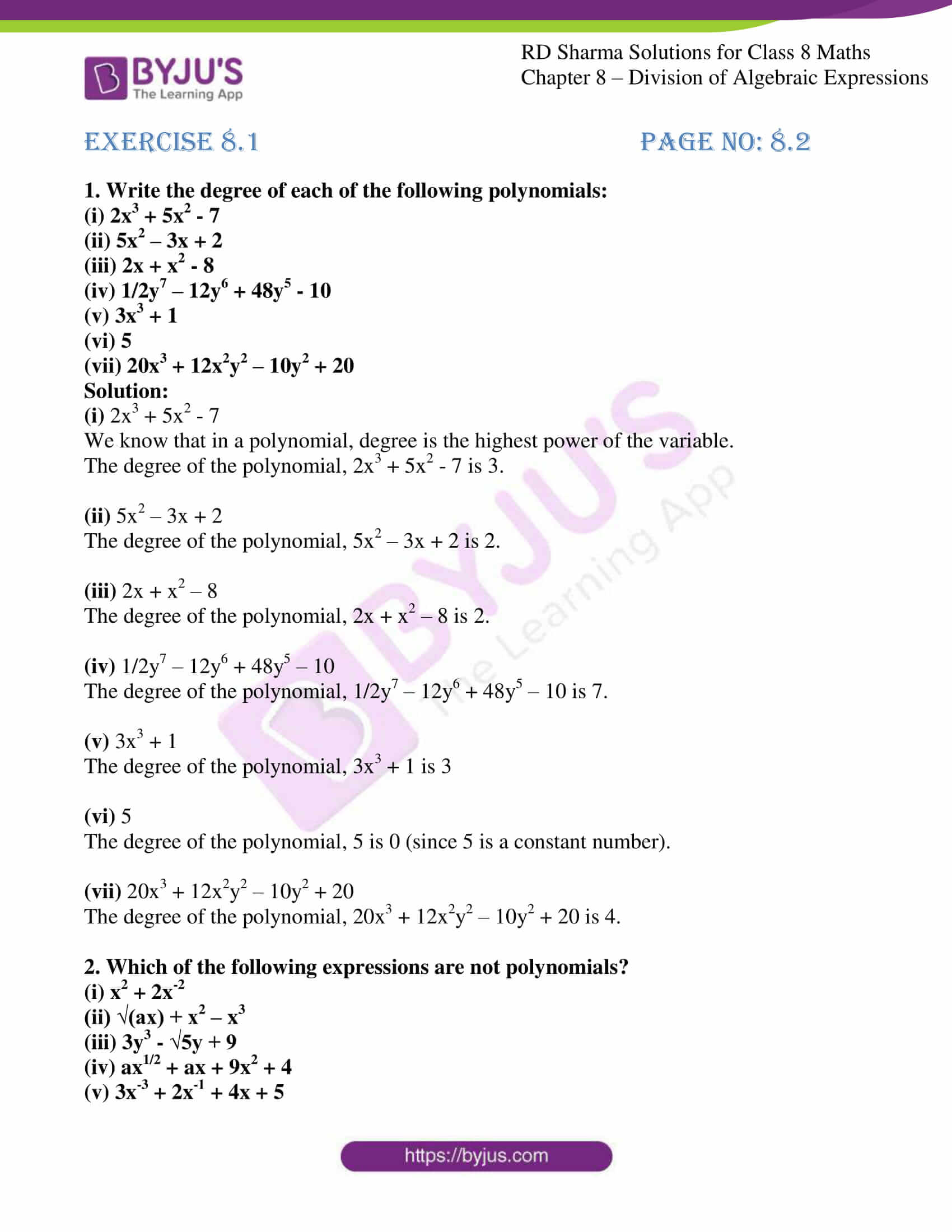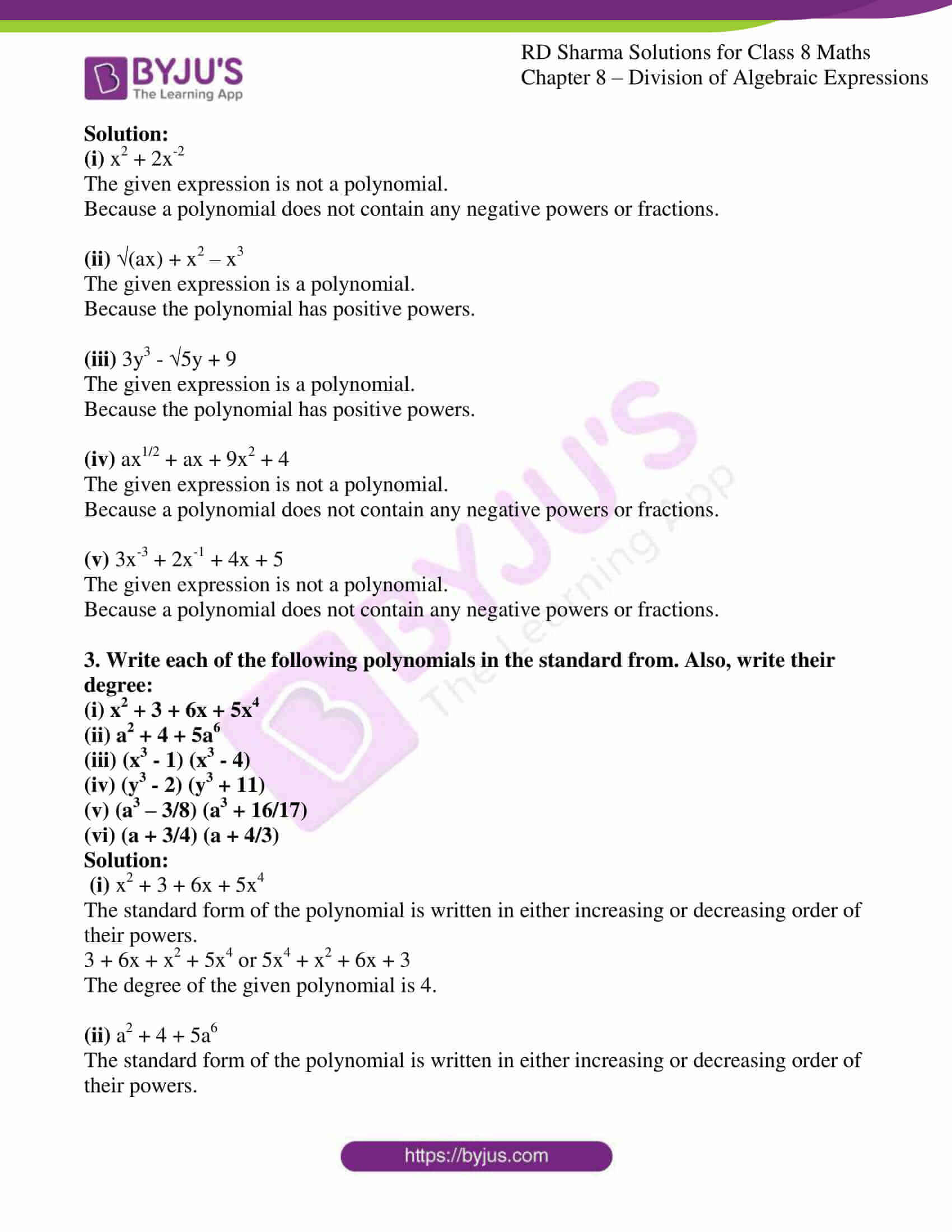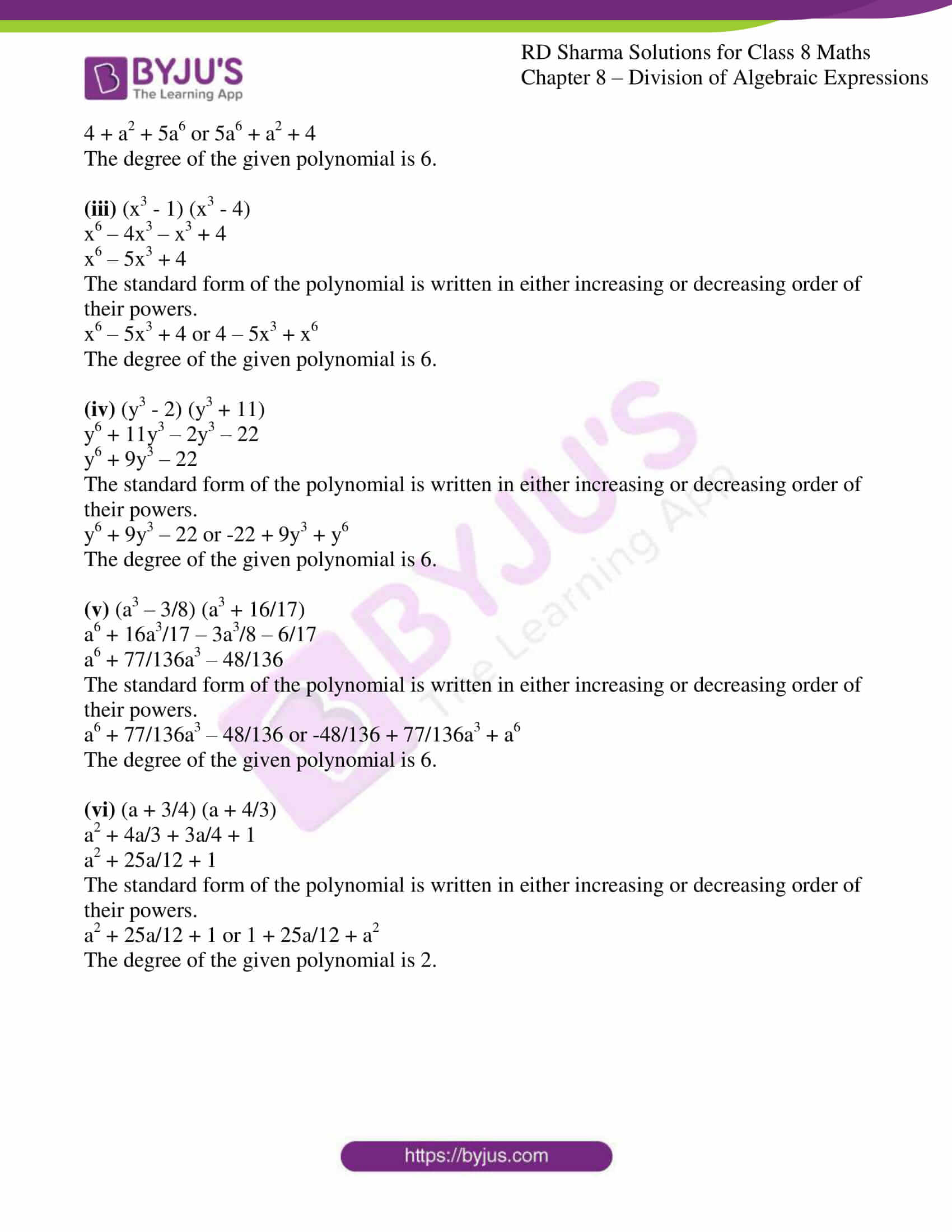# RD Sharma Solutions for Class 8 Maths Chapter 8 Division of Algebraic Expressions Exercise 8.1

The first exercise of this Chapter provides basic definitions to the terms used in this chapter, we also find the degree of a polynomial in one variable and two variables. BYJU’S expert team has designed the solutions for RD Sharma Class 8 Maths Chapter 8 to help students prepare for their exams at ease. RD Sharma Solutions is one of the best reference materials for CBSE students. Learners can download the pdf from the links provided below.

## Download the pdf of RD Sharma Solutions for Class 8 Maths Exercise 8.1 Chapter 8 Division of Algebraic Expressions### Access Answers to RD Sharma Solutions for Class 8 Maths Exercise 8.1 Chapter 8 Division of Algebraic Expressions

#### EXERCISE 8.1 PAGE NO: 8.2

1. Write the degree of each of the following polynomials:

(i) 2x3 + 5x2 – 7

(ii) 5x2 – 3x + 2

(iii) 2x + x2 – 8

(iv) 1/2y7 – 12y6 + 48y5 – 10

(v) 3x3 + 1

(vi) 5

(vii) 20x3 + 12x2y2 – 10y2 + 20

Solution:

(i) 2x3 + 5x2 – 7

We know that in a polynomial, degree is the highest power of the variable.

The degree of the polynomial, 2x3 + 5x2 – 7 is 3.

(ii) 5x2 – 3x + 2

The degree of the polynomial, 5x2 – 3x + 2 is 2.

(iii) 2x + x2 – 8

The degree of the polynomial, 2x + x2 – 8 is 2.

(iv) 1/2y7 – 12y6 + 48y5 – 10

The degree of the polynomial, 1/2y7 – 12y6 + 48y5 – 10 is 7.

(v) 3x3 + 1

The degree of the polynomial, 3x3 + 1 is 3

(vi) 5

The degree of the polynomial, 5 is 0 (since 5 is a constant number).

(vii) 20x3 + 12x2y2 – 10y2 + 20

The degree of the polynomial, 20x3 + 12x2y2 – 10y2 + 20 is 4.

2. Which of the following expressions are not polynomials?

(i) x2 + 2x-2

(ii) √(ax) + x2 – x3

(iii) 3y3 – √5y + 9

(iv) ax1/2 + ax + 9x2 + 4

(v) 3x-3 + 2x-1 + 4x + 5

Solution:

(i) x2 + 2x-2

The given expression is not a polynomial.

Because a polynomial does not contain any negative powers or fractions.

(ii) √(ax) + x2 – x3

The given expression is a polynomial.

Because the polynomial has positive powers.

(iii) 3y3 – √5y + 9

The given expression is a polynomial.

Because the polynomial has positive powers.

(iv) ax1/2 + ax + 9x2 + 4

The given expression is not a polynomial.

Because a polynomial does not contain any negative powers or fractions.

(v) 3x-3 + 2x-1 + 4x + 5

The given expression is not a polynomial.

Because a polynomial does not contain any negative powers or fractions.

3. Write each of the following polynomials in the standard from. Also, write their degree:

(i) x2 + 3 + 6x + 5x4

(ii) a2 + 4 + 5a6

(iii) (x3 – 1) (x3 – 4)

(iv) (y3 – 2) (y3 + 11)

(v) (a3 – 3/8) (a3 + 16/17)

(vi) (a + 3/4) (a + 4/3)

Solution:

(i) x2 + 3 + 6x + 5x4

The standard form of the polynomial is written in either increasing or decreasing order of their powers.

3 + 6x + x2 + 5x4 or 5x4 + x2 + 6x + 3

The degree of the given polynomial is 4.

(ii) a2 + 4 + 5a6

The standard form of the polynomial is written in either increasing or decreasing order of their powers.

4 + a2 + 5a6 or 5a6 + a2 + 4

The degree of the given polynomial is 6.

(iii) (x3 – 1) (x3 – 4)

x6 – 4x3 – x3 + 4

x6 – 5x3 + 4

The standard form of the polynomial is written in either increasing or decreasing order of their powers.

x6 – 5x3 + 4 or 4 – 5x3 + x6

The degree of the given polynomial is 6.

(iv) (y3 – 2) (y3 + 11)

y6 + 11y3 – 2y3 – 22

y6 + 9y3 – 22

The standard form of the polynomial is written in either increasing or decreasing order of their powers.

y6 + 9y3 – 22 or -22 + 9y3 + y6

The degree of the given polynomial is 6.

(v) (a3 – 3/8) (a3 + 16/17)

a6 + 16a3/17 – 3a3/8 – 6/17

a6 + 77/136a3 – 48/136

The standard form of the polynomial is written in either increasing or decreasing order of their powers.

a6 + 77/136a3 – 48/136 or -48/136 + 77/136a3 + a6

The degree of the given polynomial is 6.

(vi) (a + 3/4) (a + 4/3)

a2 + 4a/3 + 3a/4 + 1

a2 + 25a/12 + 1

The standard form of the polynomial is written in either increasing or decreasing order of their powers.

a2 + 25a/12 + 1 or 1 + 25a/12 + a2

The degree of the given polynomial is 2.